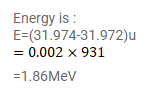# 32P beta-decays to 32S. Find the sum of the energy of the antineutrino and

Question:

${ }^{32} \mathrm{P}$ beta-decays to ${ }^{32} \mathrm{~S}$. Find the sum of the energy of the antineutrino and the kinetic energy of the $\beta$ - particle. Neglect the recoil of the daughter nucleus. Atomic mass of ${ }^{32} \mathrm{P}=31.974 \mathrm{u}$ and that of ${ }^{32} \mathrm{~S}=31.972 \mathrm{u}$.

Solution: